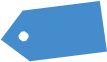# Building Blocks Part III (by The Director)

The Pareto principle, and the true distribution of the marketThe Pareto principle is one of the most important empirical properties in nature.

Fundamentally, it represents that of a near infinite variety of individual elements, most elements will be extremely small, and only a small proportion will consist of large units or have a large impact.This can be seen in nature:

1) That most grains of sand are tiny, with occasional larger grains
2) That the size of human settlements are generally small with a few exceptions
3) Size of space meteorites
4) Price returns on individual instruments
5) 80% of the productivity of a company is driven by 20% of its employees
6) And the distribution of wealth is controlled by a very tiny few

And so on…

We can graph this idea – that clustering of results of common small changes is the rule, with occasional very large exceptions.

Here is a second example, where we see that in Brownian motion (Band 1) there is a smooth, rather constant expression of events, while a representation of clustering with occasional large outliers (consistent with the Pareto principle, Band 2) is a graph of something called the Levy distribution. These patterns are distinct. Band 3 is another Brownian motion graph, while 5 and 6 represent trading of IBM and the Deutschmark exchange rates. The other bands are fictional data.Notice, that of variances in the Dow, the effect in 1987 of a 20 sigma move (Mandelbrot 2004).Normal distributions as defined in the previous post, are a specific example of a stable distribution. This is defined as a random variable X is said to have a “stable distribution” if for any n= or >2, there is a positive number Cn and a real number Dn such that
X1+X2+X3… +Xn-1 +Xn = Cn * X +Dn

Graphically, this means that the larger representation of a pattern, when zoomed in, will look extremely similar to the larger pattern. It _is_ the same pattern, only transformed by a constant. If that sounds familiar, it probably is, because the same principle is seen in the Mandelbrot set:Notice that zoomed in the pattern looks identical to the larger pattern. The Mandelbrot pattern has been seen extensively in nature, in tree branches, ice patterns, and in the human body in the intricacies of the bronchioles of the lung and in circulatory systems and capillaries.
The normal distribution and Levy distribution are both specific examples of the stable distribution. However, in a normal distribution, the curves tail off with exponential decay. That means an extreme value (say 0.15% top percentile) will represent three standard deviations away from the mean. In a normal distribution there is *fixed variance*.

In a Levy distribution, variance is infinite. In reality, trading results mirror the Pareto principle more than that of fixed variance. Most results tend to cluster in the middle, while a few, rare, huge events, occur occasionally. The data is compatible then, with *infinite variance*. This can be represented by the Levy distribution, where the tails asymptote to a value higher than 0, and large moves that would be 5 or 10 standard deviations in a normal distribution, are much smaller than this in Levy distributions.

Notice too, that the “middle” portion of a Levy distribution is narrower than the normal distribution. In the below curve, black (with alpha of 2) represents a normal distribution, while a Levy distribution (alpha 1.5) is colored in blue. The green and red curves are more extreme versions of the same Levy principle.Here are the implications.

1) Selling naked calls or puts is potentially fatal to one’s trading career, and potentially able to bankrupt any investing firm.

2) The middle proportion of the curve in reality is smaller than the normal distribution. Thus, the Black Scholes values overstate volatility. This can be taken advantage of by selling higher percentiles of volatility for an advantage. Empirically, overall, selling volatility for a Black Scholes probability of 68% is in actuality an 81% bet. A credit received for selling the volatility done over time with many occurrences will have an edge during times of higher volatility. So long as risk is capped, this is an underappreciated edge.

3) Per Mandelbrot: The larger picture is identical to the smallest figure. That means counterparties to trades, no matter how big of an institution (mid sized bank, Bank of America, Deutsche Bank, AIG, Lloyd’s, the US Federal Reserve) can be vulnerable to lethal risk, with *infinite variance*. This is extremely scary. There is no such thing as a “too big to fail”, even the US Fed. In nature, there are some waves (“perfect storm”) NO SHIP can tolerate, even the largest US aircraft carrier. There are rare extremes of turbulence that will destroy *any aircraft* no matter how large or durable. Fortunately, per the Pareto principle, these events are very very rare.

Last, the distribution of volatility of the SPX can be visualized. Notice how similar this distribution is to a Pareto distribution.(sources: Ivan Hristov slideset “Fractals in Financial Markets”, Mandelbrot 2004, Barabasi)